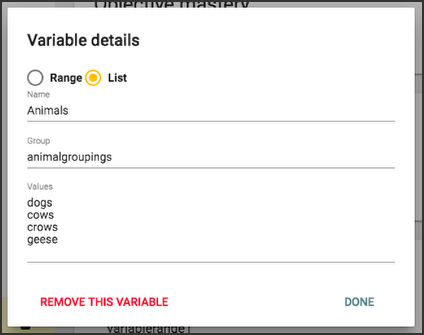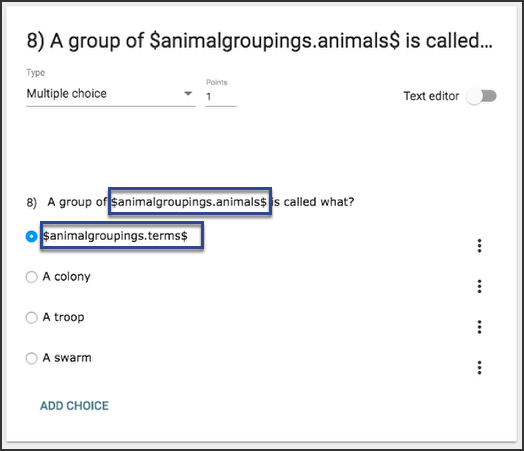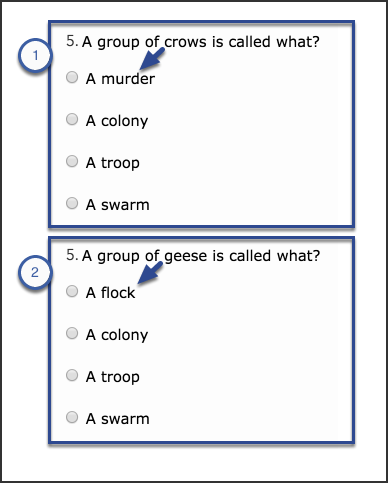# Teacher

## How do I use assessment variables?

Follow

Assessment variables allow you to create questions that use different values for each student, presenting them with varied questions and answers.

You can create two kinds of assessment variables in Buzz: ranges and lists.

## Variable ranges

Variable range values are always numbers. When you create a variable range, you provide a minimum and maximum number. When you use that variable range, Buzz randomly generates a number using those parameters.

### Create a variable range

To create a variable range:

1. Create an assessment.
2. Create an assessment question.
3. Click Add variable on the question's Variables card.1. Select Range and:
• Name the variable range (don't use spaces or special characters).
• Provide the lowest number you want included in the range in the Min field (you can use decimals and negative numbers).
• Provide the highest number you want included in the range in the Max field (you can use decimals and negative numbers).
• Provide the increments between Min and Max that you want included in the Step field. For example, if you specify 1, every whole within the parameters could be used. If you specify 2, only every other whole number can be used (you can use decimals).
2. Click Done.### Use a variable range

Once you have created a variable range, you can use it in questions and answer options.

Example

This example uses two variable ranges in a multiple choice question. Here's how they're used:

1. Place the variable range Name between dollar signs (\$) to signal Buzz to use the variable range:
``\$variablerange1\$``
1. Within a question, Buzz generates the same number for every instance of the variable range. This allows you to create answer options that use the same number that's generated in the question.
2. You can create math problems using variable range and have Buzz show the solution by:
• Typing the math problem in parentheses.
• Adding \$eval in front of the parenthesis.
• Adding a comma and a number sign (#) the end of the problem, inside the parentheses.
``\$eval(\$variablerange1\$+\$variablerange2\$,#)``
1. You can indicate how many decimal places you'd like included by adding a decimal and the desired number of places as zeros after the number sign (#) and inside the parentheses.This question is now generated differently for each assessment attempt, as in the two instances shown here.## Variable lists

Variable lists allow you to create a set of items, each of which can be swapped in at random anywhere you want in a question.

### Create a variable list

To create a variable list:

1. Create an assessment.
2. Create an assessment question.
3. Click Add variable on the question's Variables card.1. Select List and:
• Name the variable list (don't use spaces or special characters).
• Provide a Group title (don't use spaces or special characters).
• Provide the Values for the items in the list in a list.
2. Click Done.### Use a variable list

Once you have created a variable list, you can use it in questions and answer options.

Example

More than one variable list can be part of the same Group, allowing you to align items between multiple variable lists in one question. To do this, you use the same Group title for multiple lists with different Names. For example, one variable list in a Group titled animalgroupings could list animals (with Animals as the Name), and a second list with the same Group title might list the terms used for groupings of those animals (with Terms as the Name). The items align by position in the list, like this:

• Name: Animals, Group: animalgroupings, Values: dogs, cows, crows, geese
• Name: Terms, Group: animalgroupings, Values: pack, herd, murder, flock

This example uses these two variable lists (Animals and Terms) as part of the same Group (animalgroupings) in a multiple choice question. To reference the variable lists in the question, you place Group.Name between dollar signs (\$), like this:

• \$animalgroupings.animals\$
• \$animalgroupings.terms\$This question is now generated differently for each assessment attempt, as in the two instances shown here. Notice that the items from each list align correctly.Sort by

Can this be applied to a fill in the blank question? If so how would you do it?

0

Hello Amy!

The same variable function is available for Fill In The Blank type questions. It will be the same steps to configure as shown in this document using the variable lists option.
Variable range values are always numbers and are especially useful for questions pertaining to math.

0

I didn't realize that underscore was considered a "special character" and so I spent a lot of time trying to figure out why my variable \$cell_count\$ wasn't working.

Instead of just silently failing, it would be nice if the system prevented you from using an incompatible name.  For example, the name box could be listening for a blur event and then scrub the string of all 'bad' characters, or it could give an alert.  It would make the user interface more friendly.  Especially since a lot of users will be using features without having read through the documentation word for word.

0

Nowhere in this documentation does it mention that the eval function works differently for fill in the blank answers than it does for visual elements.

From what I can tell (based on trial and error).

If it is a visible number -->  \$eval(formula,#)

If it is a fill in the blank answer --> eval(formula)

You might want to mention this somewhere.  Or if it is documented elsewhere can you direct me to that page?

0

Hey Drhoades, you are correct. We need to update our documentation in this site to include some of these more advanced assessment operations. In the meantime, you may find this page helpful.

0

@Drhoades something else to be aware of (that I also discovered the hard way) with

If it is a visible number -->  \$eval(formula,#)

If the calculated value is 0 it won't be displayed. The # is a blank space that is filled with the calculated value (unless it is 0).

If you use -->  \$eval(formula,0)

Then it uses 0 as a placeholder. The 0 is replaced by the calculated value. If the calculated value is 0 then 0 is displayed.

Also, are you aware that you need to use a decimal and however many places you want it if the displayed value is a decimal?

If it is a visible number with a decimal -->  \$eval(formula,#.###)

0

Is there a way for the values inside of a list variable to reference other variables?  Or to run evals?

Simple Fill in the Blank Example:

a = \$a\$

b = \$b\$

What is the \$math.operation\$ of A and B ?

***

Variables:

a = 5..9

b= 2..4

operation(math) = [ sum , difference , product , quotient ]

answer(math) = [ eval(\$a\$+\$b\$) , eval(\$b\$-\$a\$) , eval(\$a\$*\$b\$) , eval(\$a\$/\$b\$) ]

0

Is there a way to reference variables from a Passage inside of the questions that are linked to that passage?  I teach Physics and I want to make story problems that have lots of range variables, and then break the solution down into separate "baby step" questions that guide the student towards the final answer.  But I can't figure out how to extend the scope of the passage variables so that I can access them inside other questions.

0

I'm not 100% sure what you are asking regarding the operations. You should certainly be able to do this though:

Var: a = 2..15

Var: b = 5..12

Var: operation = +,-,*,/

1) Here, in the question you could display `\$a\$ \$operation\$ \$b\$`.  Notice that I put that inside accent marks to make it a math expression so it will display nicer. You could also have it display the evaluated answer `\$eval(\$a\$ \$operation\$ \$b\$,#.###)`

You could also tie it to the operation name with a list variable like this:

Var: a = 2..15

Var: b = 5..12

Var: op.eration = +,-,*,/

Var: op.name = sum, difference, product, quotient

2) Now in the question you could say... Find the \$op.name\$: `\$a\$ \$operation\$ \$b\$`

\$op.name\$ = _______________

a. eval(\$a\$ \$operation\$ \$b\$)

Is that anything like you were asking?

0

Bruce

In this particular example I don't want the student to see which math symbol ( + - * / ) they should use because I am testing their vocabulary.  But you are right that by putting the symbol into a list variable I can dynamically create the answer key.  I will have to play around with cutting my list variables into smaller pieces to see if this will work on some of my other questions as well.

0

Interesting idea for a question. So you're thinking something like the question below (but likely more complex). You can copy it and paste it into your course to try it out.

Type: F, Match
Options: Multiple
Score: 2, Partial
Var: a = 12..25
Var: b = 2..12,2
Var: a.ddname = sum,difference
Var: a.ddop = +,-
Var: m.ultname = product,quotient
Var: m.ultop = *,/
1) Write the expression represented by the phrase below; then evaluate it.
The \$a.ddname\$ of \$a\$ and the \$m.ultname\$ of \$b\$ and 2.
expression: __________________
evaluated: __________________
[0.5]a. \$a\$ \$a.ddop\$ \$b\$ \$m.ultop\$ 2
[0.5]b. eval(\$a\$\$a.ddop\$\$b\$\$m.ultop\$2)

0

Bruce -- Yes that is the general idea for my previous example.  The equation itself is dynamic.  Your idea works well for this example, but there are other times where I really wish I could "nest" one variable inside another.

Do you have any ideas on an easier way to do this type of question, for example.

*********

My variables are:

A,B,C,D,E = 50..100 , 5

F = 75..85 , 5

q.letter = [ A , B , C , D , E ]

The answer is mathematically simple:  take the student score and subtract the class average

The first thing I tried to do was "nest" the variables like this:  eval(\$\$q.letter\$\$-\$F\$)

I was hoping that \$q.letter\$ would return the letter choice --> A and then the eval would run: eval(\$A\$-\$F\$)

But it didn't work.

So then I tried making a list variable to hold the range variables:  q.score = [ \$A\$ , \$B\$ , \$C\$ , \$D\$ , \$E\$ ]   where the answer was eval(\$q.score\$-\$F\$)

I was hoping that \$q.score\$ would return the desired variable --> \$A\$ and then the eval would run:  eval(\$A\$-\$F\$)

But this didn't work either.

I'm pretty sure the "find and replace" function they are running on their eval string only runs once and so it misses the nested variable.

I did come up with an alternate method.  But it is cumbersome.  I have had to create five extra variables to eliminate unwanted numbers from my formula:

q.A2 = [ 1 , 0 , 0 , 0 , 0 ]

q.B2 = [ 0 , 1 , 0 , 0 , 0 ]

q.C2 = [ 0 , 0 , 1 , 0 , 0 ]

q.D2 = [ 0 , 0 , 0 , 1 , 0 ]

q.E2 = [ 0 , 0 , 0 , 0 , 1 ]

And then my answer key is eval((\$A\$*\$q.A2\$+\$B\$*\$q.B2\$+\$C\$*\$q.C2\$+\$D\$*\$q.D2\$+\$E\$*\$q.E2\$)-\$F\$)

But as you can imagine, as the formulas get more complicated this "elimination" method gets really ugly really fast.  Any other ideas?

I know I could reduce the variability.  For example, always ask about Student C.  But I want to pack as much variation into a single question as I possibly can.

0

I tried to use nested variables once too and couldn't find a way for it to work. It would certainly enable a lot more options. It would also be great if we could somehow work in simple if..then commands too. Sorry I can't be of more help.

0

There should be a modding (%) function.

I am trying to create a question where I need 5/3=2 and I use the eval function w/ no decimals (\$eval(\$var1\$/\$var2\$,#)) but the function rounds! Going to have to try and code around this, especially since you can't make variables depending on other variables.

Sorry for ranting, I just thought there would be more math functions available, like spreadsheets.

0

Can variables do any kind of a word counter? For example I want my students to write a paragraph. (I will use variables for key words, but I would also like it to reject answers of less than 150 words--or characters if that works but words do not).

0

@Amy, no, variables would not do any word counter. By "reject," do you mean you just want them to have a minimum count before they can submit or would you want to flunk it automatically if less than 150 words?

0

Regarding a word count, it would be really useful if you could set a word count that somehow alerted the student when their answer was "long enough".  (For example, the outline of the box changed colors.) And if they did try to submit the assessment with a "too short" response they would get an alert that says, "One or more of your responses was too short.  Are you sure you want to submit?"  And then answers that are too short don't even get graded.  They are just an automatic zero.  This would be very useful for "participation" type questions that the teacher doesn't want to grade themselves.  Because then they could use RegEx to check for target words and use word count to ensure that the student had taken the question seriously.

0

I would love it if it just rejected an answer and said this answer is not long enough.

0